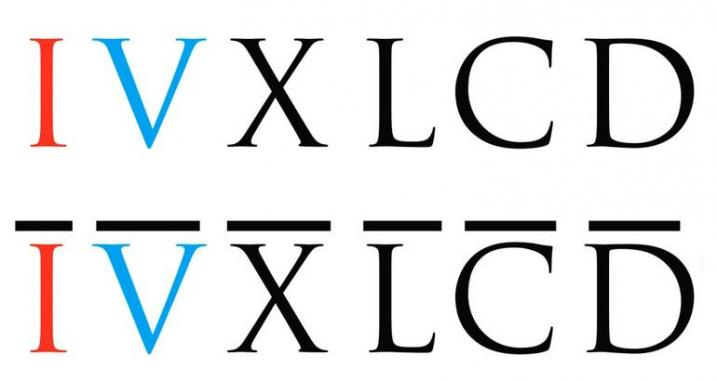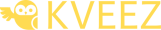Knowledge is power, so learn.

# Roman numerals

Roman numerals are a numeral system that was used by ancient Rome. Numbers in this system are represented by combinations of letters from the Latin alphabet.

Roman numerals were in use until the 14th century, when system was replaced by the more convenient Arabic numerals. However, Roman numerals are still used today in certain contexts.## Roman numerals Converter

With Roman numerals converter you can convert numbers between 1 and 3999. For larger numbers Romans used identical letters with a line above the letter.

Enter a Roman numeral and convert it to a decimal.

Enter a Roman numeral

{{ronumdn}} = {{ronumres}}

Enter a decimal number and convert it to a Roman numeral.

Enter a decimal number

{{arnumdn}} = {{arnumres}}

## Learn Roman numerals

Select a Roman numeral and check out its decimal value.

{{shwro}} = {{shwar}}

## Interactive exercises for converting Roman numerals

Interactive exercises are generic, which means that the application compiles a new series of questions each time you play.
Before start choose a number and a difficulty of the questions.{{acttit}}
{{doque.id+1}}. question
{{doque.ans.f1}} =

{{doque.id+1}}. question
{{doque.ans.f1}} =

{{doque.id+1}}. question
{{doque.ans.f1}} =

{{doque.id+1}}. question
{{doque.ans.f1}} =

{{doque.id+1}}. question
{{doque.ans.f1}} =

{{doque.id+1}}. question
{{doque.ans.f1}} =

{{doque.id+1}}. question
{{doque.ans.f1}} =

{{doque.id+1}}. question
{{doque.ans.f1}} =

{{doque.id+1}}. question
{{doque.ans.f1}} =

{{doque.id+1}}. question
{{doque.ans.f1}} =

{{doque.id+1}}. question
{{doque.ans.f1}} =

{{doque.id+1}}. question
{{doque.ans.f1}} =

{{doque.id+1}}. question
{{doque.ans.f1}} =

{{doque.id+1}}. question
{{doque.ans.f1}} =

{{doque.id+1}}. question
{{doque.ans.f1}} =Result - {{acttit}}
{{anscor}}
{{answro}}

Practice makes perfect.

START QUIZ{{quitits}}
DIFFICULTY
NUMBER OF QUESTIONS
START QUIZ{{quitits}}
DIFFICULTY
NUMBER OF QUESTIONS
START QUIZ
SHARE WITH FRIENDS
If you like our quizzes and learning apps, please share them on social networks.

Thanks, have a nice day.
POPULAR
KNOWLEDGE CARDS

## Table Roman numerals

Basic table for converting Roman numerals into Arabic decimals.

 Roman numerals Arabic numerals I 1 V 5 X 10 L 50 C 100 D 500 M 1000

Roman numerals have no symbol to represent 0.

## Roman numerals conversion rules

If a numeral comes after a numeral that is larger or equal in value, then it must be added to the numeral before it.
VI = 5 + 1 = 6
XX = 10 + 10 = 20

2. Subtraction
A smaller numeral placed before a larger numeral indicates subtraction of that smaller numeral from the larger one.
You cannot subtract more than one value from a Roman numeral.
IV = 5 - 1 = 4
IX = 10 - 1 = 9

3. Max three together
You cannot add more than three of the same Roman numeral together.
III = 3
Wrong: IIII, correct: IV = 4

4. L and D cannot be subtracted
Only powers of ten (I, X, C, M) can be subtracted.
Value 5
Wrong: VX (10 - 5)
Correct: V (5)

5. One-fifth or one-tenth
You can subtract only value that is 1/5th (one-fifth) or 1/10th (one-tenth) the larger one.
Wrong: IC = 100 - 1 = 99
Correct: XCIX = (100 - 10) + (10 - 1) = 99

POPULAR
REVIEWS
To submit a review please LOGIN. Do not have profile yet? CREATE PROFILE.